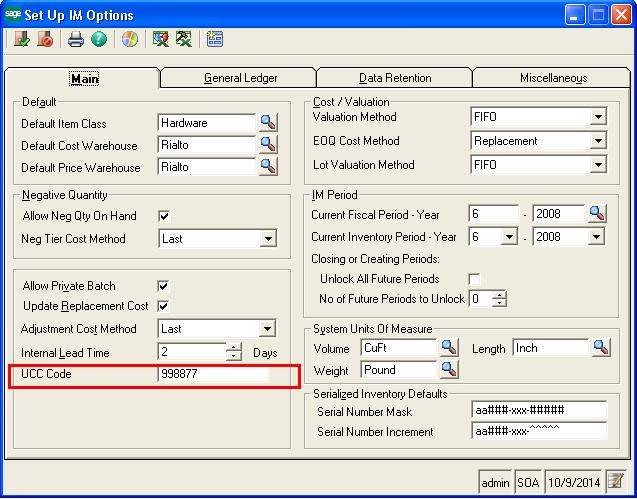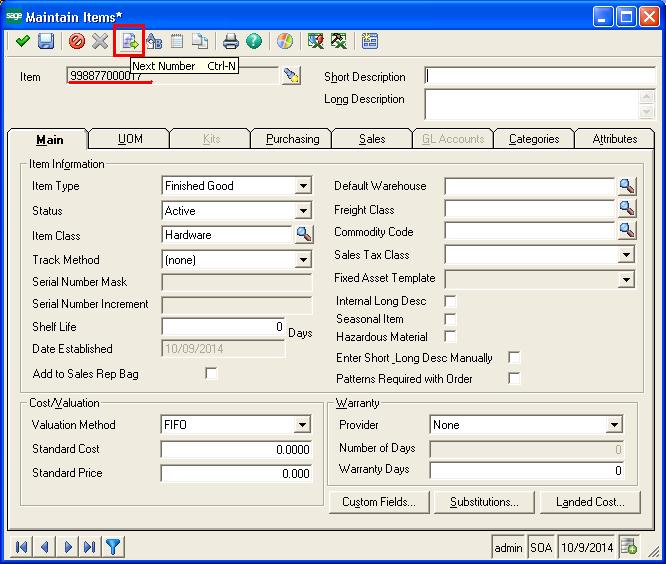# UPC Code generated at Item Master in Sage 500

By | February 23, 2017

There was a very unique but interesting requirement from one of our clients recently; the requirement is the new Item id need to be generated automatically by using Universal Product Code generation logic in Sage 500 Erp.

New StuffVendor Return Flow

Basically, the Universal Product Code is the unique 12- digit number and the purpose is to make item id easily identify the product features like brand, style, color, and size. The UPC code always helpful in tracking inventory, Maintain Barcode and Fixed asset Management.  The barcode scanner can immediately scan the UPC Code item id and identify the product to provide all the information quickly. Also, improve the efficiency and reliability while tracking the inventory.

The formula of UPC Code generation is very unique which is a combination of UCC Code + Product Seq No + Algorithm no to check digit calculation.

UPC Code formula (12 digit) = UCC Code (6 digit) + Product Seq No (5 digit) + Check Sum will be auto-calculated by using algorithm no to check digit calculation (1 digit)

• UCC Code (6 digit no): A uniform commercial code is first published in 1952, is one of the 6 digit numbers which is generally used while commercial transactions. Our client was fixed 6 digit UCC code: 998877.
• Product Seq. no. (5 digit no): This sequence no we have auto-generated based on the item generation history. Example: “00001”  – 1st Item, “00002” – 2nd Item,”00003” – 3rd Item
• The checksum will be auto-calculated by using algorithm no to check digit calculation (1 digit) : This formula basically uses for determining what number needs to be added.

Check Sum Calculation Logic:

Let’s assume that we are using the fictitious code 05432122345.

1. Add all of the digits in the odd positions (digits in position 1, 3, 5, 7, 9, and 11)  0 + 4 + 2 + 2 + 3 + 5 = 16
2. Multiply by 3.   E.g.   16 * 3 = 48
3. Add all of the digits in even positions (digits in position 2, 4, 6, 8 and 10).   5 + 3 + 1 + 2 + 4 = 15
4. Sum the results of steps 3 and 2.  E.g. 48 + 15 = 63
5. Determine what number needs to be added to the result of step 4 in order to create a multiple of 10.  E.g.   63 + 7 = 70
6. The check digit therefore equals 7.

Finally, using this formula we need to generate the output item id code: UPC Code = 998877000017 UPC CODE (998877000017) = UCC Code (998877) + Product Seq no (00001) + Checksum digit no (7)

To achieve this requirement, what we did we incorporated the UCC Code with textbox into the Setup IM Options which is fixed 6 digit no “998877”.Then, we have introduced new next number button on the Maintain Items Screens to generate the UPC code. Whenever user clicks to next number button our customization has been followed all calculation formula of UPC code and generate the UPC code no. 998877000017.

This way we managed the UPC code generation logic and incorporated into the Maintain Items screen.

It would be interesting to know if this kind of functionality is required by others as well.